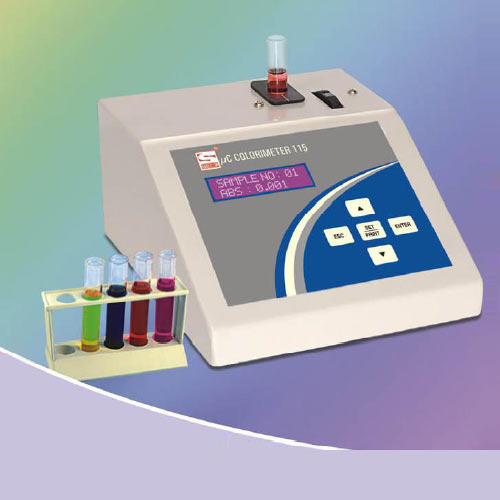# Colorimetry Viva

Q.1 What is Lambert’s law?

Lambert‘s law states that the proportion of light absorbed by an absorbing substance is independent of the intensity of the incident light.

Q.2 What is Beer’s law?

Beer’s law states that the proportion of light absorbed depends only on the total number of absorbing molecules through which light passes.

Q.3 Define photometry.

Photometry is the most common analytical technique used in clinical biochemistry.

Principle of photometry is based on the physical laws of radiant energy. The intensity of absorbed transmitted or reflected light is measured and is related to the concentration of the test substrate.

Q.4 Define colorimetry.

This is a technique of measurement, i.e. quantitative analysis of substance in all biological fluids. Basis of doing this is to convert the substance into a coloured products by performing various specific reactions. The intensity of color is directly proportional to the amount of substance present in the sample.

Q.5. What is optical density?

Optical density (OD) is the logarithmic ratio of the intensity of the incident light to that of the emergent light.

OD = log10 Io/I

where,

Io is the intensity of incident light.

I is the intensity of emergent light.

Q.6 What is transmission?

Transmission is defined as the ratio of the intensity of the transmitted light to that of the incident light.

T = I/Io

where,

Io is the intensity of incident light.

I is the intensity of emergent light.

Q.7. What is Lambert-Beer’s law?

Lambert-Beer’s law states that when monochromatic light passes through a colored solution, the amount of light transmitted decreases exponentially.

1. With a decrease in thickness of the layer of solution through which the light passes.
2. With an increase in concentration of the colored substance.

The relation is

I/Io = e-ktc

Where, I = intensity of emergent light.

Io = intensity of incident light.
k = a constant.
c = concentration of the colored substance.
t = thickness of the layer of the solution

Q.8 What is the relation between the optical density and transmittance?

Optical density and transmittance are related by the formula.

Optical density = 2–log (Transmittance)

Q.9 Define standard curve.

When OD or absorbance on Y-axis is plotted against con-centration on X-axis, a straight line passing through the origin is obtained which means Beer’s law is followed.

With the help of a standard curve, the concentration of the unknown can be readily determined.

Q.10 What is the use of blank in colorimetric estimation?

The function of the bank is to eliminate the effect of light absorption by the regents used, since otherwise they might lead to falsely high values for optical density and consequently to falsely high values for the concentrations of the substance it is desired to determine.

Q.11 What are complementary colors?

 Colors of light Complementary colors Violet Yellow-green Blue-green Orange Blue Yellow Green-blue Red Green Purple/red Yellow Blue